# New to QlikView

Discussion board where members can get started with QlikView.

New Contributor III

## Handle Null Dimension in Pivot Table

Hi All,

I got a pivot table as below where product #bbb contain null time bucket.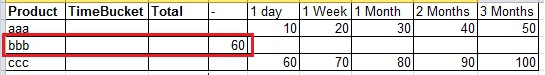I got request to suppress the value of null time bucket for product #bbb by manual assign (60 * 40%) into 1 Week & (60 * 60%) into 2 Months time bucket as below.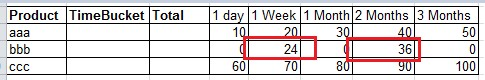Appreciate if anyone can help to provide solution.

Thanks,

Boon Haw

9 RepliesMVP

## Re: Handle Null Dimension in Pivot Table

May be like this:

If(Product = 'bbb', if(TimeBucket = '1 week', Sum(TOTAL <Product> Measure) * 0.40, If(TimeBucket = '2 months', Sum(TOTAL <Product> Measure) * 0.60)), Sum(Measure))MVP

## Re: Handle Null Dimension in Pivot Table

Here is a sample for you to look at:New Contributor III

## Re: Handle Null Dimension in Pivot Table

Hi Sunny,

Thanks a lot for your effort.

From the expression below, the product is set as #bbb, in case there are a lot of product and have no idea which product has null time bucket. How can we find out which product has null time bucket.

If(Product = 'bbb', if(TimeBucket = '1 Week', Sum(TOTAL <Product> Sales) * 0.40, If(TimeBucket = '2 Months', Sum(TOTAL <Product> Sales) * 0.60, 0)), Sum(Sales))MVP

## Re: Handle Null Dimension in Pivot Table

You can try this:

If(Sum({<TimeBucket = {'*'}>}Value) > 0, Sum(Value),

If(TimeBucket = '1 Week', Sum(TOTAL <Product> Value) * 0.40,

If(TimeBucket = '2 Months', Sum(TOTAL <Product> Value) * 0.60, 0)))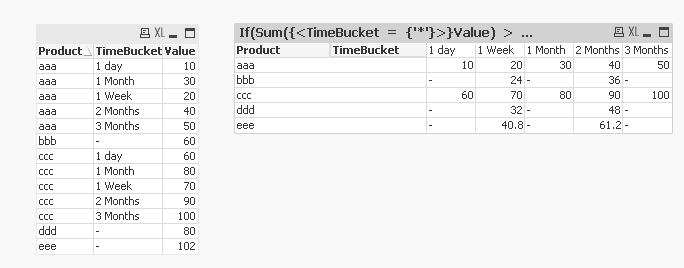New Contributor III

## Re: Handle Null Dimension in Pivot Table

Hi Sunny, Thanks a lot for your effortMVP

## Re: Handle Null Dimension in Pivot Table

No problem at all. Does this give you what you were looking for? If it did, would you be able to close this thread by marking correct and any helpful responses?

Qlik Community Tip: Marking Replies as Correct or Helpful

Best,

Sunny

New Contributor III

## Re: Handle Null Dimension in Pivot Table

Hi Sunny,

Recently the user request to show partial sums in pivot.

But unfortunately the total value show "-".

Would need your help to check if can show the partial sums value for product bbb.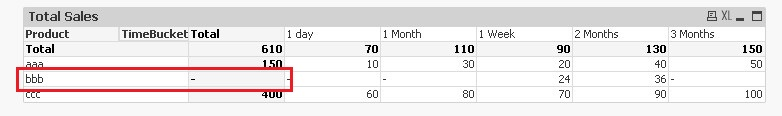MVP

## Re: Handle Null Dimension in Pivot Table

Try this:

If(Product = 'bbb' and SecondaryDimensionality() = 1 and Dimensionality() = 1,

If(TimeBucket = '1 Week', Sum(TOTAL <Product> Value) * 0.40,

If(TimeBucket = '2 Months', Sum(TOTAL <Product> Value) * 0.60, 0)), Sum(Value))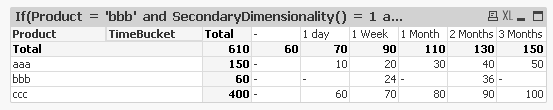New Contributor III

## Re: Handle Null Dimension in Pivot Table

Thanks a lot Sunny, the solution work perfectly.